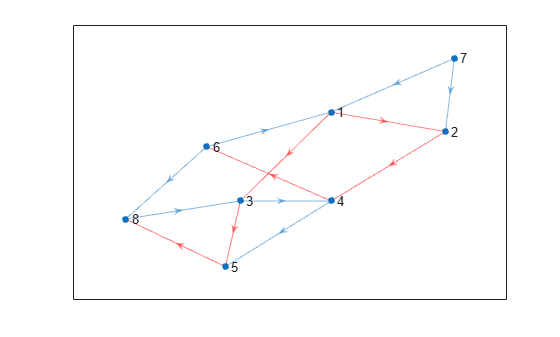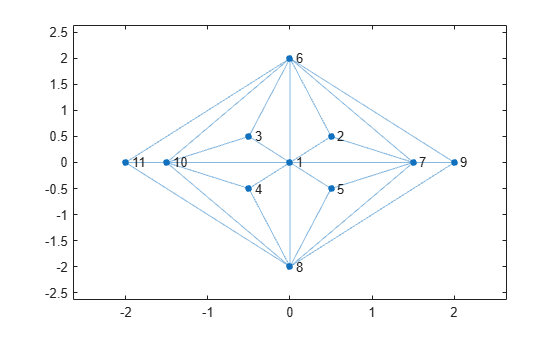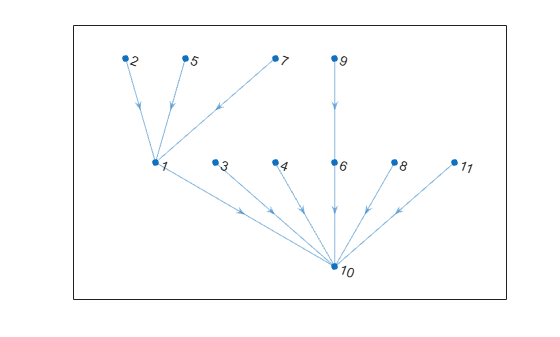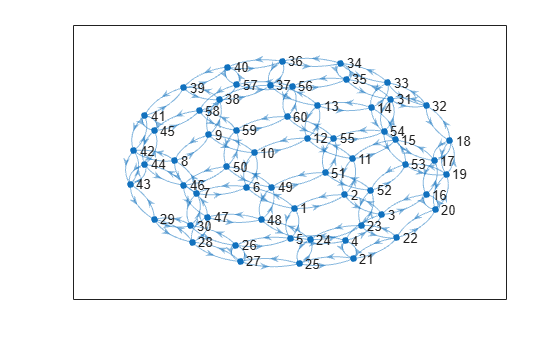Documentation

### This is machine translation

Mouseover text to see original. Click the button below to return to the English version of the page.

# shortestpathtree

Shortest path tree from node

## Syntax

``TR = shortestpathtree(G,s)``
``TR = shortestpathtree(G,s,t)``
``TR = shortestpathtree(___,Name,Value)``
``````[TR,D] = shortestpathtree(___)``````
``````[TR,D,E] = shortestpathtree(___)``````

## Description

example

````TR = shortestpathtree(G,s)` returns a directed graph, `TR`, that contains the tree of shortest paths from source node `s` to all other nodes in the graph. If the graph is weighted (that is, `G.Edges` contains a variable `Weight`), then those weights are used as the distances along the edges in the graph. Otherwise, all edge distances are taken to be `1`.```

example

````TR = shortestpathtree(G,s,t)` computes the tree of shortest paths between multiple source or target nodes:`s` can be a single source node, and `t` can specify multiple target nodes.`s` can specify several source nodes, and `t` can specify a single target node.```

example

````TR = shortestpathtree(___,Name,Value)` uses additional options specified by one or more Name-Value pair arguments, using any of the input argument combinations in previous syntaxes. For example, `shortestpathtree(G,s,'OutputForm','vector')` returns a numeric vector that describes the shortest path tree.```

example

``````[TR,D] = shortestpathtree(___)``` additionally returns the shortest path distance between nodes in the tree.```
``````[TR,D,E] = shortestpathtree(___)``` additionally returns a logical vector `E` that indicates whether each graph edge is in `TR`.```

## Examples

collapse all

Find the shortest paths from a source node to each of the other reachable nodes in a graph, and plot the results.

Create a directed graph.

```s = [1 1 2 3 3 4 4 6 6 7 8 7 5]; t = [2 3 4 4 5 5 6 1 8 1 3 2 8]; G = digraph(s,t)```
```G = digraph with properties: Edges: [13x1 table] Nodes: [8x0 table] ```

Calculate the shortest paths from node 1 to each of the other reachable nodes in the graph. Then, plot the resulting tree on top of the graph.

```TR = shortestpathtree(G,1); p = plot(G); highlight(p,TR,'EdgeColor','r')```Since there is no path from node 1 to node 7, node 7 is disconnected from the tree.

Find the shortest paths from each node in a graph to a target node, and plot the results.

Create and plot a graph.

```s = [1 1 1 1 1 1 1 2 2 7 7 7 7 9 9 3 3 1 6 4 8 10 6 8 4 5]; t = [2 3 4 5 6 8 7 6 7 5 6 8 9 6 8 6 10 10 10 10 10 11 11 11 8 8]; G = graph(s,t); x = [0 0.5 -0.5 -0.5 0.5 0 1.5 0 2 -1.5 -2]; y = [0 0.5 0.5 -0.5 -0.5 2 0 -2 0 0 0]; plot(G,'XData',x,'YData',y)```Find the shortest paths from each node in the graph to node 10. Plot the resulting tree.

```TR = shortestpathtree(G,'all',10); plot(TR)```Find the shortest paths and path lengths from a single source node to several target nodes.

Create and plot a graph.

```G = digraph(bucky); plot(G)```Find the shortest paths from node 23 to several other nodes. Specify `OutputForm` as `cell` to return the shortest paths in a cell array. Specify two outputs to also return the shortest path distances.

```target = [1 5 13 32 44]; [TR,D] = shortestpathtree(G,23,target,'OutputForm','cell')```
```TR = 5x1 cell array {1x6 double} {1x5 double} {1x8 double} {1x6 double} {1x6 double} ```
```D = 1×5 5 4 7 5 5 ```

`tree{j}` is the shortest path from node 23 to node `target(j)` with length `D(j)`.

Find the path and path length from node 21 to node 5.

`path = TR{2}`
```path = 1×5 23 22 21 4 5 ```
`path_length = D(2)`
```path_length = 4 ```

## Input Arguments

collapse all

Input graph, specified as either a `graph` or `digraph` object. Use `graph` to create an undirected graph or `digraph` to create a directed graph.

Example: `G = graph(1,2)`

Example: `G = digraph([1 2],[2 3])`

Source node(s), specified as one or more node indices or node names, or as all nodes in the graph with `'all'`.

• When used alone, `s` must specify a single source node.

• When used together with `t`, the `s` and `t` inputs must satisfy:

• `s` can be a single source node, and `t` can specify multiple target nodes.

• `s` can specify several source nodes, and `t` can specify a single target node.

This table shows the different ways to refer to one or more nodes either by their numeric node indices or by their node names.

FormSingle NodeMultiple Nodes
Node index

Scalar

Example: `1`

Vector

Example: `[1 2 3]`

Node name

Character vector

Example: `'A'`

Cell array of character vectors

Example: `{'A' 'B' 'C'}`

String scalar

Example: `"A"`

String array

Example: `["A" "B" "C"]`

`s` must not specify the node name `'all'`, since this node name conflicts with an option name. Use `findnode` to instead pass in the node index for this case.

Example: `shortestpathtree(G,'a')`

Example: `shortestpathtree(G,[1 2 3],8)`

Target node(s), specified as one or more node indices or node names, or as all nodes in the graph with `'all'`.

The `s` and `t` inputs must satisfy:

• `s` can be a single source node, and `t` can specify multiple target nodes.

• `s` can specify several source nodes, and `t` can specify a single target node.

`t` must not specify nodes named `'all'`, `'Method'`, or `'OutputForm'`, since these node names conflict with option names. Use `findnode` to instead pass in the node index for these cases.

Example: `shortestpathtree(G,[1 2 3],8)`

Example: `shortestpathtree(G,{'a','b','c'},{'f'})`

### Name-Value Pair Arguments

Specify optional comma-separated pairs of `Name,Value` arguments. `Name` is the argument name and `Value` is the corresponding value. `Name` must appear inside quotes. You can specify several name and value pair arguments in any order as `Name1,Value1,...,NameN,ValueN`.

Example: ```[TR,D] = shortestpathtree(G,s,t,'Method','unweighted','OutputForm','vector')```

Format of output, specified as the comma-separated pair consisting of `'OutputForm'` and one of the options in the table.

OptionDescription
`'tree'` (default)

`TR` is a directed graph representing the shortest path tree. If specified, the third output `E` is a logical vector indicating whether each edge is in `TR`.

`'cell'`

`TR` is a cell array, and `TR{k}` contains the path from `s` to `t(k)` or from `s(k)` to `t`. If there is no path between the nodes, then `TR{k}` is empty.

If `s` and `t` are node names, then `TR{k}` is a cell array of character vectors. Otherwise, `TR{k}` is a numeric vector.

If specified, the third output `E` is a cell array indicating the edges on each corresponding path in `TR`.

`'vector'`

`TR` is a vector that describes the tree:

• If `s` contains a single source node, then `TR(k)` is the ID of the node that precedes node `k` on the path from `s` to `k`. By convention, `TR(s) = 0`.

• If `s` contains multiple source nodes, then `TR(k)` is the ID of the node that succeeds node `k` on the path from `k` to `t`. By convention, `TR(t) = 0`.

In each case `TR(k)` is `NaN` if node `k` is not part of the tree.

If specified, the third output `E` is a vector where `E(k)` gives the index of the edge of the shortest path tree connecting node `k` and node `TR(k)`.

Example: `shortestpathtree(G,s,'OutputForm','vector')`

Shortest path algorithm, specified as the comma-separated pair consisting of `'Method'` and one of the options in the table.

OptionDescription
`'auto'` (default)

The `'auto'` option automatically selects the algorithm:

• `'unweighted'` is used for `graph` and `digraph` inputs with no edge weights.

• `'positive'` is used for all `graph` inputs that have edge weights, and requires the weights to be nonnegative. This option is also used for `digraph` inputs with nonnegative edge weights.

• `'mixed'` is used for `digraph` inputs whose edge weights contain some negative values. The graph cannot have negative cycles.

`'unweighted'`

Breadth-First computation that treats all edge weights as `1`.

`'positive'`

Dijkstra algorithm that requires all edge weights to be nonnegative.

`'mixed'` (only for `digraph`)

Bellman-Ford algorithm for directed graphs that requires the graph to have no negative cycles.

While `'mixed'` is slower than `'positive'` for the same problem, `'mixed'` is more versatile as it allows some edge weights to be negative.

`'acyclic'` (only for `digraph`)

Algorithm designed to improve performance for directed, acyclic graphs (DAGs) with weighted edges.

Use `isdag` to confirm if a directed graph is acyclic.

### Note

For most graphs, `'unweighted'` is the fastest algorithm, followed by `'acyclic'`, `'positive'`, and `'mixed'`.

Example: `shortestpath(G,s,t,'Method','acyclic')`

## Output Arguments

collapse all

Shortest path tree, returned as a `digraph` object, cell array, or vector, depending on the value of `'OutputForm'`. Use the `highlight` function to visualize the shortest path tree on top of a plot of the graph, or use `plot(TR)` to visualize the shortest path tree on its own.

If there are multiple shortest paths between two nodes, then `TR` contains only one of the paths. The path that is returned can change depending on which algorithm is specified by `Method`. The `TR` output is a graph with zero edges if there are no paths connecting any of the specified nodes.

Distance between source and target nodes, returned as a vector. A value of `Inf` indicates there is no path between two nodes.

Edges in tree or on path, returned as a logical vector, cell array, or vector, depending on the value of `'OutputForm'`:

• If you don't specify `'OutputForm'` or specify a value of `'tree'`, then `E` is a logical vector indicating whether each graph edge is in directed graph `TR`. This output is compatible with the `'Edges'` name-value pair of `highlight`, for example: `highlight(p,'Edges',E)`.

• If `'OutputForm'` is `'cell'`, then `E` is a cell array containing the edges on the corresponding paths in `TR`.

• If `'OutputForm'` is `'vector'`, then `E` is a vector which, for each node, gives the index of the edge connecting it to its parent node in the shortest path tree.

## Tips

• The `shortestpath`, `shortestpathtree`, and `distances` functions do not support undirected graphs with negative edge weights, or more generally any graph containing a negative cycle, for these reasons:

• A negative cycle is a path that leads from a node back to itself, with the sum of the edge weights on the path being negative. If a negative cycle is on a path between two nodes, then no shortest path exists between the nodes, since a shorter path can always be found by traversing the negative cycle.

• A single negative edge weight in an undirected graph creates a negative cycle.